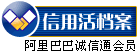# 1 问题的提出图3 普通外圆车刀的干涉

# 2 车刀的走刀轨迹分析

 N100 M06 TO101; 01号刀具为圆弧刀具 N110 G00 X22 Z-16; 快速接近圆弧起始点 N120 GO1 X20 Z-16 F200 S500; 进给到圆弧的起始点 N130 G02 X20 Z-34 I12 K-9: 顺时针加工圆弧 N140 G01 X22 Z-34; 径向退刀图4 轴类零件的圆弧形轮廓图5 尖刀加工圆弧型面图6 尖刀干涉分析图图7 圆弧刀加工原理图

∵DE、00'均为垂直于Z轴的直线∴DE//00'

∴D0'//E0
D0'=E0〔平行四边形对应边平行且相等)
∴DO'=EO=R-r

# 3 起点与终点的确定

sin∠A0P=AP/A0=|Xa-X0|/R(Xa、X0为A点和0点的X轴坐标)
A'Q=A'0sin∠AOP=(R-r)sin∠AOP=(R-r)|Xa-X0|/R
X'0=X0A'Q=X0(R-r)|Xa-X0|/R
Xm=Xa-r=X0(R-r)[|Xa-X0|/R]-r
cos∠A0P=0P/A0=|Za-Z0|/R(Za、Z0为A点和0点的Z轴坐标)
PQ=AA'cos∠AOP=|Za-Z0|/R

∴Xm=18.8Z，Zm=-17.8；Xn=18.8；Zn=-32.2

 N100 M06 T0202; 02号刀具为所选圆弧刀具 N110 GOO G90 X21 Z-17.8 M03; 快速进给到指定位置 N120 GO1 X18.8 Z-17.8 F100; 径向进给圆弧起始点 N130 G02 X18.8 Z-32.2 R12; 加工圆弧 N140 GO1 X21 Z-32.2; 径向退刀

# 4 结束语